# ​ Use appropriate algebra and Theorem 7.2.1 to find the given inverse Laplace transform.

Use appropriate algebra and Theorem 7.2.1 to find the given inverse Laplace transform. (Write your answer as a function of t.)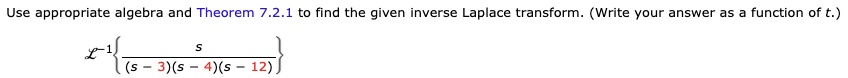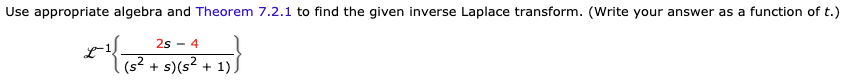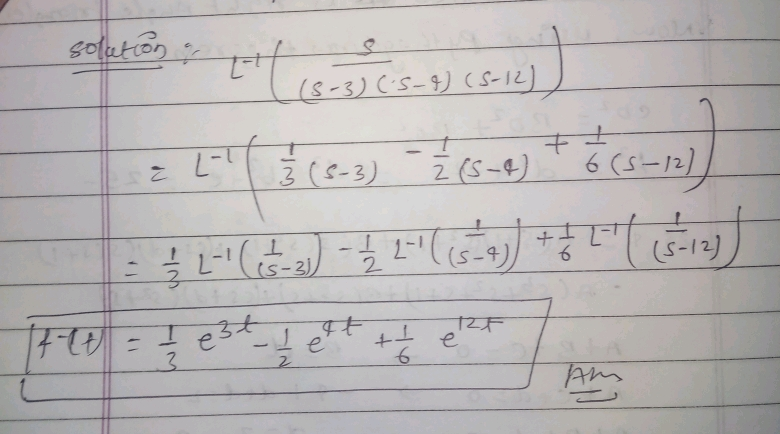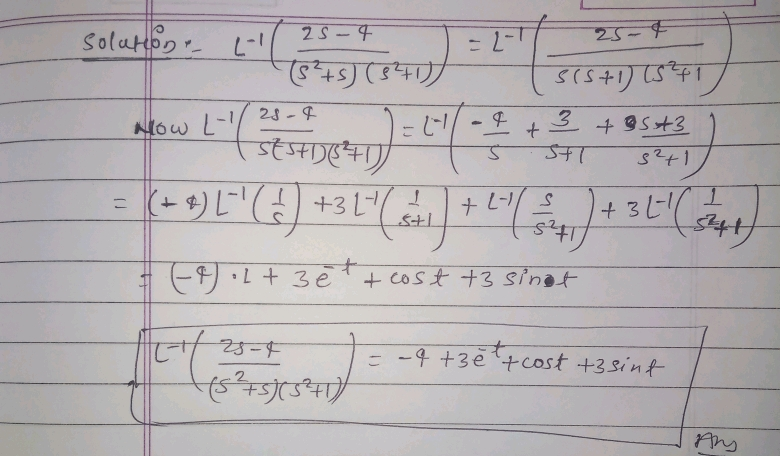#### Earn Coin

Coins can be redeemed for fabulous gifts.

Similar Homework Help Questions
• ### Use appropriate algebra and Theorem 7.2.1 to find the given inverse Laplace transform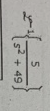Use appropriate algebra and Theorem 7.2.1 to find the given inverse Laplace transform. (Write your answer as a function of t.)

• ### Use appropriate algebra and Theorem 7.2.1 to find the given inverse Laplace transform. (Write your answer...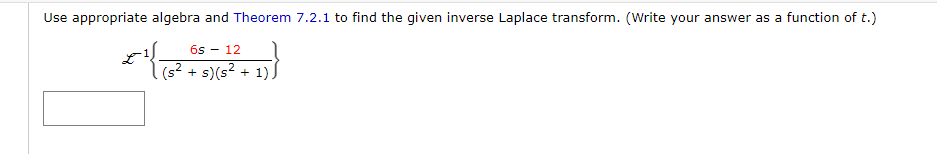Use appropriate algebra and Theorem 7.2.1 to find the given inverse Laplace transform. (Write your answer as a function of t.) ℒ−1 6s − 12 (s2 + s)(s2 + 1) Use appropriate algebra and Theorem 7.2.1 to find the given inverse Laplace transform. (Write your answer as a function of t.) il 65 - 12 (s2 + s) (s2 + 1)

• ### 1)Use appropriate algebra and Theorem 7.2.1 to find the given inverse Laplace transform. (Write your answer...

1)Use appropriate algebra and Theorem 7.2.1 to find the given inverse Laplace transform. (Write your answer as a function of t.) ℒ−1 8 s2 + 49 2) Use appropriate algebra and Theorem 7.2.1 to find the given inverse Laplace transform. (Write your answer as a function of t.) ℒ−1 9s 9s2 + 1

• ### Use appropriate algebra and Theorem 7.2.1 to find the given inverse Laplace transform. (Write your answer...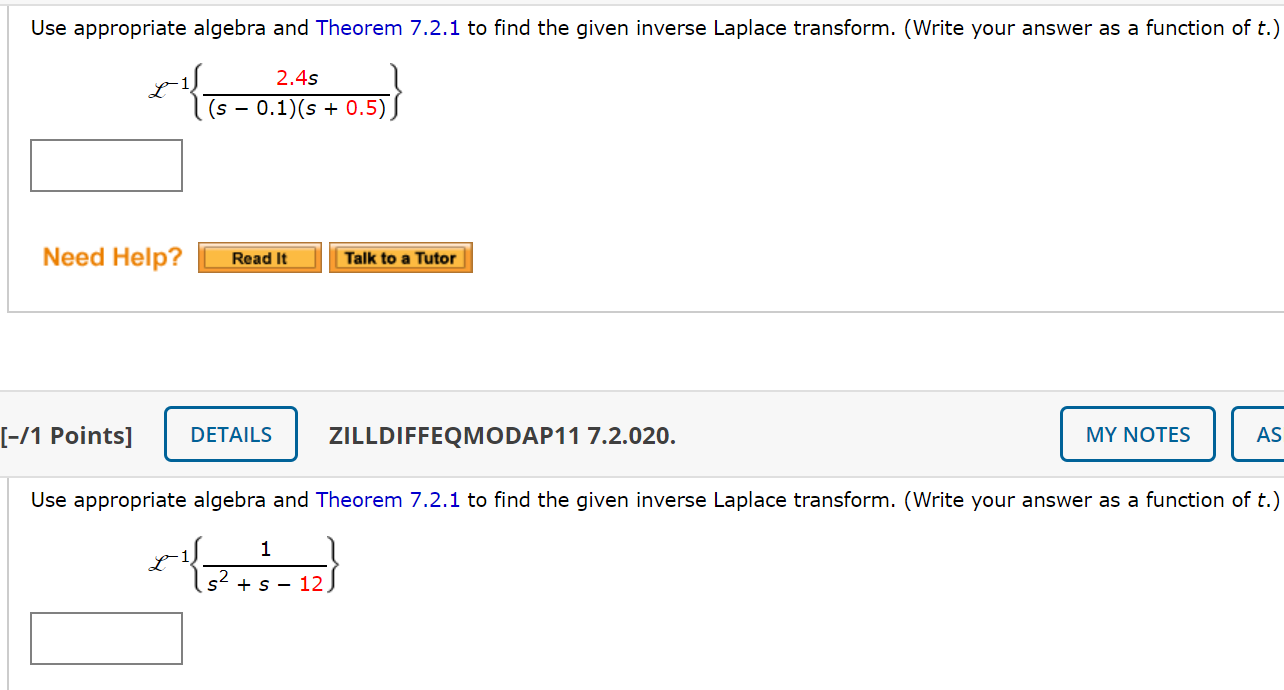Use appropriate algebra and Theorem 7.2.1 to find the given inverse Laplace transform. (Write your answer as a function of t.) 2.4s (s - 0.1)(s + 0.5)) Need Help? Read It Talk to a Tutor [-/1 Points] DETAILS ZILLDIFFEQMODAP11 7.2.020. MY NOTES AS Use appropriate algebra and Theorem 7.2.1 to find the given inverse Laplace transform. (Write your answer as a function of t.) 1 s2 + S - 12

• ### please answer all three Use appropriate algebra and Theorem 7.2.1 to find the given inverse Laplace...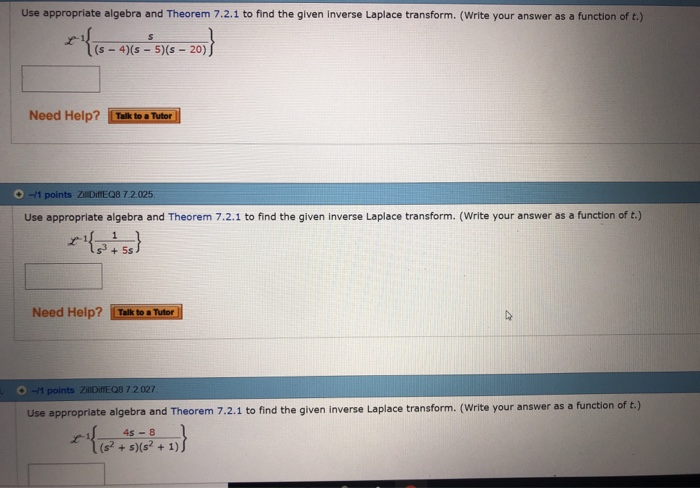please answer all three Use appropriate algebra and Theorem 7.2.1 to find the given inverse Laplace transform. (Write your answer as a function of t.) x{15-45-515–201} Need Help? Talk to a Tutor 0-1 points ZillDiffEQB 7.2.025 Use appropriate algebra and Theorem 7.2.1 to find the given inverse Laplace transform. (Write your answer as a function of t.) Need Help? Talk to Tutor 0-1 points ZDE08 7 2 027 Use appropriate algebra and Theorem 7.2.1 to find the given inverse Laplace...

• ### Use appropriate algebra and Theorem 7.2.1 to find the given inverse Laplace transform.(Write your answer as...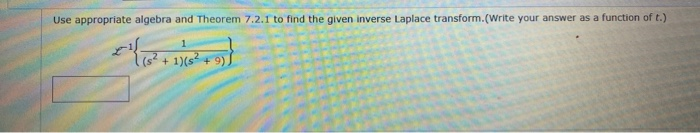Use appropriate algebra and Theorem 7.2.1 to find the given inverse Laplace transform.(Write your answer as a function of t.) + 1)(s2 + 9)

• ### Use appropriate algebra and Theorem 7.2.1 to find the given inverse Laplace transform. (Write your answer...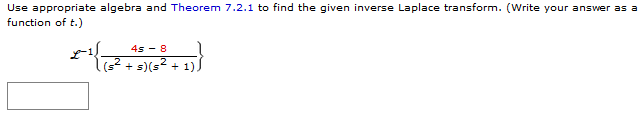Use appropriate algebra and Theorem 7.2.1 to find the given inverse Laplace transform. (Write your answer as a function of t.) 4s-8 (s2s)s2

• ### Use appropriate algebra and Theorem 7.2.1 to find the given inverse Laplace transform. (Write your answer...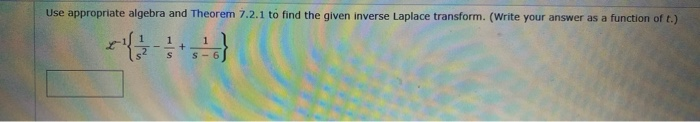Use appropriate algebra and Theorem 7.2.1 to find the given inverse Laplace transform. (Write your answer as a function of t.) 1 + 1 S - 6 S

• ### Use appropriate algebra and Theorem 7.2.1 to find the given inverse Laplace transform. (Write your answer...

Use appropriate algebra and Theorem 7.2.1 to find the given inverse Laplace transform. (Write your answer as a function of t.) ℒ−1 1/(5s + 1)

• ### Use appropriate algebra and Theorem 7.2.1 to find the given inverse Laplace transform. (Write your answer...

Use appropriate algebra and Theorem 7.2.1 to find the given inverse Laplace transform. (Write your answer as a function of t.) ℒ−1 1 s2 + s − 12